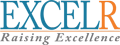# Data Science Interview Questions### myMock Interview Service for Real Tech Jobs## Neural Networks

### 1. What is a Neural Network?

Ans:- Neural Network is a supervised machine learning algorithm which is inspired by human nervous system and it replicates the similar to how human brain is trained. It consists of Input Layers, Hidden Layers, & Output Layers.

### 2. What are the various types of Neural Networks?

Ans:- Artificial Neural Network, Recurrent Neural Networks, Convolutional Neural Networks, Boltzmann Machine Networks, Hopfield Networks are examples of the Neural Networks. There are a few other types as well.

### 3. What is the use of activation functions in neural network?

Ans:- Activation function is used to convert a input signal of a node in a A-NN to an output signal. That output signal now is used as a input in the next layer in the stack.

### 4. What are the different types of activation functions in neural network?

Ans:- Sigmoid or Logistic, Tanh or Hyperbolic tangent, ReLu or Rectified Linear units are examples of activation functions in neural network

### 5. What is the package name to implement neural network in R?

Ans:- neuralnet package can be used to implement neural network in R

### 6. What is Cluster Sampling?

Ans :-It is a process of randomly selecting intact groups within a defined population, sharing similar characteristics. Cluster Sample is a probability sample where each sampling unit is a collection or cluster of elements.